Open In App
Related Articles

# Laws of Reflection

Laws of Reflection is a principle or rule that governs the phenomenon of reflection of light. The law of reflection states that a light ray will reflect off a surface at the same angle that it hit it when it comes into contact with it. The angle at which a light beam strikes a surface is known as the angle of incidence, and the angle at which the beam of light bounces off the surface is known as the angle of reflection. You can always determine how a light ray will behave, i.e., what direction it will go in and at what angle, by observing a single light ray approach and bounce off a flat mirror. In general, this is how the law of reflection works.

Now let us learn more about the Laws of reflection by understanding the concepts like What are the Laws of reflection? its formula, verification of laws of reflection, Laws of reflection for plane and rough surfaces, and examples of them.

## Reflection of Light

The process due to which the light rays fall on the surface, and get bounced back is known as a reflection of light. Light is recognized for its highly predictable behaviour. The behaviour of a ray of light as it reflects would satisfy a definite law known as the law of reflection if it could be seen approaching and reflecting off of a flat mirror. The law of reflection is shown in the diagram below.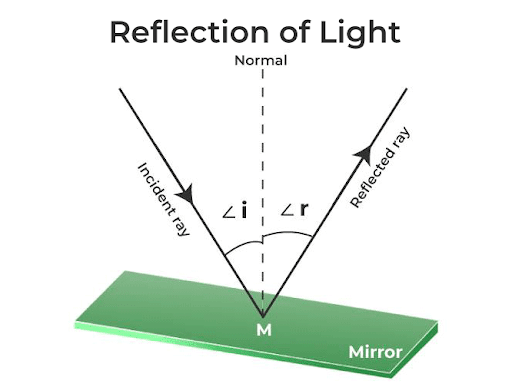There are several terms associated with the reflection of light which is as follows:

### Incident Ray

The ray of light that straightaway falls on the reflecting surface from the object or a source is known as an incident ray.

### Reflected Ray

The ray of light which is supposed to be reflected back after striking the reflecting surface is known as the reflected ray.

### Angle of Incidence

The angle that is formed between the incident ray and the general at the point of incidence is known as the angle of incidence, and it is generally represented by ∠i.

### Angle of Reflection

The angle that is formed between the reflected ray and the general at the point of incidence is known as the angle of reflection,  and it is generally represented by ∠r.

### Normal

A straight line perpendicular to the reflecting surface that acts as a separation between the plane of incidence and the plane of reflection is known as the normal.

## Types of Reflection

There are two types of Reflection, which are as follows:

• Regular Reflection
• Diffused Reflection

### Regular Reflection​

This reflection type is created by plane mirrors with smooth surfaces. The image formed is precise and sharp. Such that, all the light rays falling on the surface are reflected equally in a uniform manner. It is also known as Specular Reflection.

### Irregular Reflection

It is referred to as uneven or diffused reflection when light reflects from a rough surface in an erratic manner. As a result, the brightness and reflection quality is diminished. It is also known as Diffused Reflection.

The image added below shows the types of reflection.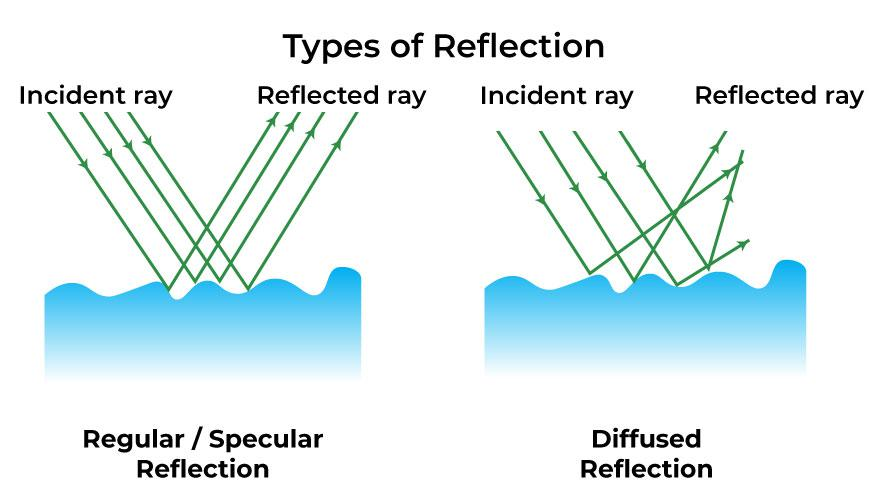## Laws of Reflection

The two laws of Reflection are,

1. The first law of reflection states that the reflection point and the point of incidence are generally comparable. The reflected light will follow the same path if the original beam strikes the plane mirror at an angle other than the usual, like 90°. Thus, the first Law of reflection state that, the angle of incidence (∠i) is always equal to the angle of reflection (∠r).
2. The second law of reflection states that the point of incidence, the incident ray, the reflected ray, and the plane of reflection are all located on the same surface.

### Laws of Reflection Formula

The Law of Reflection Formula is the term used for the essential relation stated in the Laws of Reflection i.e. the relationship between the angle of reflection and the angle of incidence.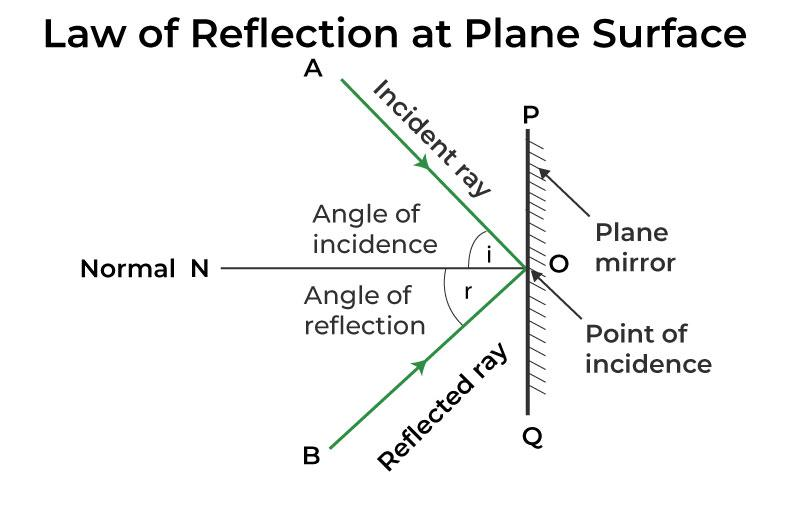The Laws of Reflection Formula can be stated as:

Angle of Incidence = Angle of Reflection

Reflected Angle = Incident Angle

∠i = ∠r

where,

• ∠i represent angle of incidence
• ∠r represents angle of reflection

### Angle of Incidence

Angle of incidence is defined as the angle made by the incident ray and the Normal. It is denoted using the ∠i.

### Angle of Reflection

Angle of reflection is defined as the angle made by the reflected ray and the Normal. It is denoted using the ∠r.

For regular reflection. i.e. reflection from the regular surface, angle of incidence is always equal to angle of reflection.

We can easily calculate the angle of incidence and the angle of reflection by drawing a normal line that is perpendicular to the reflecting surface.

## Experimental Verification of the Laws of Reflection

The laws of reflection can be verified by the following experiment, as shown in the figure below: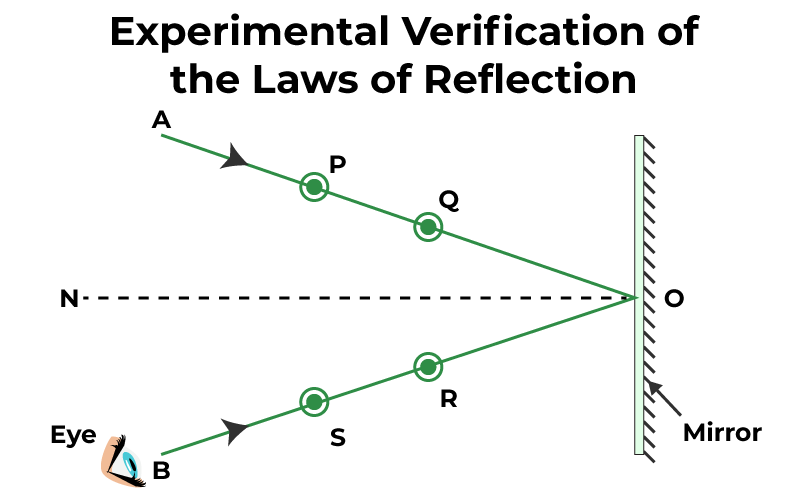• Consider a plane sheet of paper, which is fixed on a flat surface, and a straight line MM1 as shown in the figure above.
• On-Line MM1 a point O is considered, and a line OA is drawn so that ∠MOA<90 degrees. So, normal ON is drawn on MM1.
• Two pins P and Q are set vertically on the path OA, at a distance from the surface. Keeping the eye on the other side of the normal the images of P and Q are clearly seen.
• A pin R is fixed such that it is in line with the images of pins P and Q as observed in the mirror. Now one more pin S is fixed such that the pin S is in line with pin R and the images of pins P and Q.
• On the paper, tiny circles are drawn around the pins’ positions. Now the pins are removed. The pinpoints S and R are connected by a line OB, which intersects the mirror’s surface at O.
• ∠AON = ∠i is the angle of incidence, and ∠BON = ∠r is the angle of reflection.
• AO is the incident ray and OB is the reflected ray. We measure the angles ∠AON and ∠BON. The angle of incidence AON is discovered to be equal to the angle of reflection BON.
• The experiment is repeated with various incident beam AO ray directions. Angles of incidence and reflection are discovered to be equal in each instance.

## Laws of Reflection at Plane Surfaces

A plane mirror refers to a mirror with a flat (planar) reflective surface. The light rays when striking a plane mirror, the angle of reflection equals the angle of incidence always. The angle of the incidence refers to the angle between the incident ray and the surface normal (an imaginary line perpendicular drawn to the surface). In a similar way, the angle of reflection is the angle between the reflected ray and the normal at the point of incidence.

### Characteristics of the Image formed by Plane Mirror

• The image formed is precise and of the same size as the object.
• The position of the image beyond the mirror is the same as that of the object in front.
• The image formed is virtual.
• The plane mirror always forms a real image of the object.
• The image obtained in the plane mirror is always erect.
• The phenomenon of lateral inversion can be observed. Where the right appears to be left and vice versa.
• If we stand in front of the mirror the image will be of the same size, height, virtual, erect, and laterally inverted.

Concave and convex mirrors are some of the widely used mirrors which come under the spherical mirrors and can produce virtual images of an object similar to a plane mirror. On the contrary side, the images formed by them are not always of the same size as the object as in the case of a plane mirror. The image by a convex mirror, the virtual image formed is always diminished in size, however in the case of a concave mirror when the object is placed somewhere between the focus and the pole of the mirror, an enlarged virtual image can be seen. However, when we need a virtual image of the same size a plane mirror is preferred over spherical mirrors.

## Laws of Reflection at Rough Surfaces

When light reflects from a rough surface in an irregular pattern, it is known as irregular or diffused reflection. Let’s use an example to better grasp this. Imagine that a wall is illuminated. Parallel light rays will not reflect in a parallel pattern. Diverse directions are where the light that reflects spreads. The law of light reflection is at work here as well.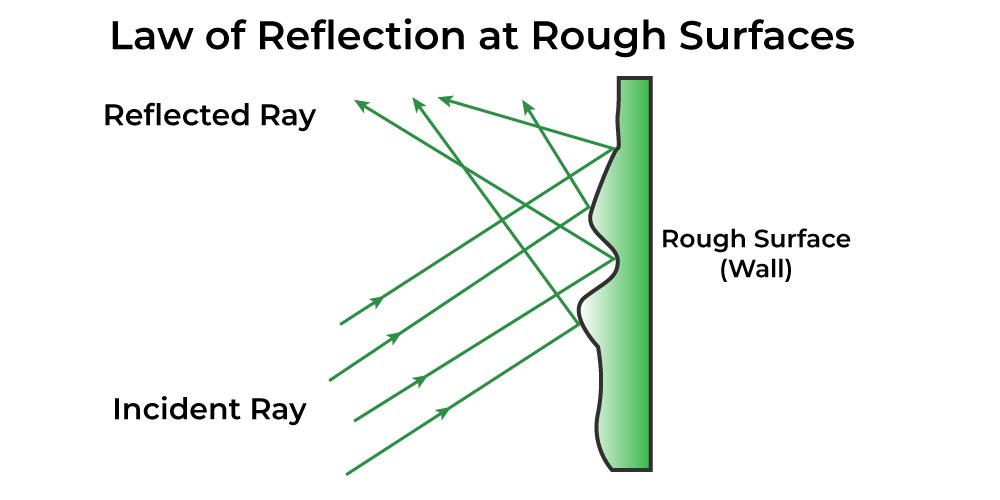## Uses of Reflection of Light

There are several use cases where the understanding of the reflection of light help, some of those use cases are as follows:

• To prevent parallax mistakes, meters like ammeters and voltmeters use mirrors. The image of the pointer is directly somewhere under the pointer when the reading is taken from that place.
• A convex and a plane mirror, respectively, are used to make a car’s wing and rearview mirrors. The driver can see objects on both sides of the car due to the two wing mirrors. The driver may see objects behind the vehicle due to the rearview mirror.
• A mirror is used in a microscope to reflect light onto the material being examined.
• Dentists also inspect a patient’s teeth using concave mirrors. The teeth appear magnified in the concave mirror.
• Torches and automobile headlamps both use parabolic mirrors as reflectors. To create parallel beams, a tiny bulb is put at the mirror’s focal point.
• As they produce magnified and upright images, concave mirrors with extended focal lengths can be used as shaving or makeup mirrors.

## Solved Examples on Laws of Reflection

Example 1. If the angle of incidence is 30 degrees then find the angle between normal and the surface.

Angle between surface and normal is always equal to 90 degree.

Example 2. What will be the angle of reflection for the incident angle of 45°?

We know that,

∠ i = ∠ r

Therefore, ∠r = 45°

Hence, the angle of reflection will be 45°

Example 3. What will be the angle of incidence for the reflected angle 40°?

We know that,

∠ i = ∠ r

Therefore, ∠i = 40°

Hence, the angle of incidence will be 40°

Example 4. What will be the incident angle if the angle between the mirror surface and the incident ray is 50°?

We know that,

∠i + angle between mirror and incident ray = 90°

Therefore, ∠i + 50° = 90°

Therefore, ∠i = 90° – 50°

Therefore, ∠i = 40°

Hence, the incident angle is 40°

## FAQs on Laws of Reflection

### Q1: What are the laws of reflection?

Laws of reflection are the fundamental laws that tells us about the motion of the light rays when it strikes a solid surface. Their are two laws of reflection that states that,

1. According to the primary law of reflection, the reflection point and the point of incidence are generally comparable. The reflected light will follow the same path if the original beam strikes the plane mirror at an angle other than the usual, like 90°. Thus, the first Law of reflection state that, the angle of incidence (∠i) is always equal to the angle of reflection (∠r).
2. The point of incidence, the incident ray, the reflected ray, and the plane of reflection are all located on the same surface.

### Q2: What is the Angle of Reflection?

The angle of reflection (∠r) is the angle between the reflected ray and the normal plane surface.

### Q3: What is the Second Law of Reflection?

The second law of reflection states that the incident ray, reflected ray, and the normal, all lie on the same plane.